# 1、简介

matplotlib 是Python可视化程序库的泰斗。经过十几年它任然是Python使用者最常用的画图库。它的设计和在1980年代被设计的商业化程序语言MATLAB非常接近。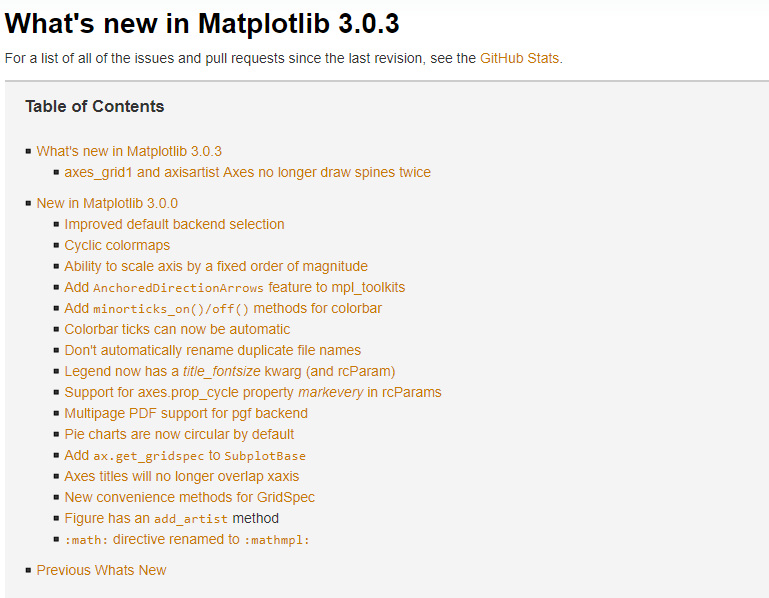Github/matplotlib：https://github.com/matplotlib/matplotlib

# 2、用 matplotlib 绘制各种可视化图表

## 2.1 折线图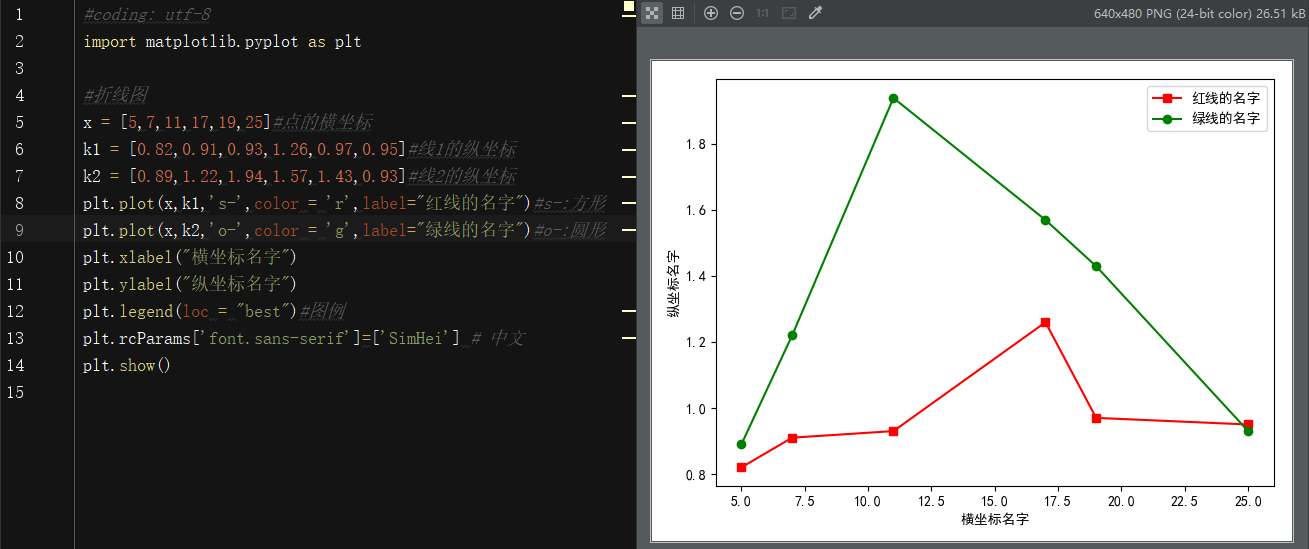#coding: utf-8
import matplotlib.pyplot as plt

#折线图
x = [5,7,11,17,19,25]#点的横坐标
k1 = [0.82,0.91,0.93,1.26,0.97,0.95]#线1的纵坐标
k2 = [0.89,1.22,1.94,1.57,1.43,0.93]#线2的纵坐标
plt.plot(x,k1,'s-',color = 'r',label="红线的名字")#s-:方形
plt.plot(x,k2,'o-',color = 'g',label="绿线的名字")#o-:圆形
plt.xlabel("横坐标名字")
plt.ylabel("纵坐标名字")
plt.legend(loc = "best")#图例
plt.rcParams['font.sans-serif']=['SimHei'] # 中文
plt.show()


## 2.2 柱形图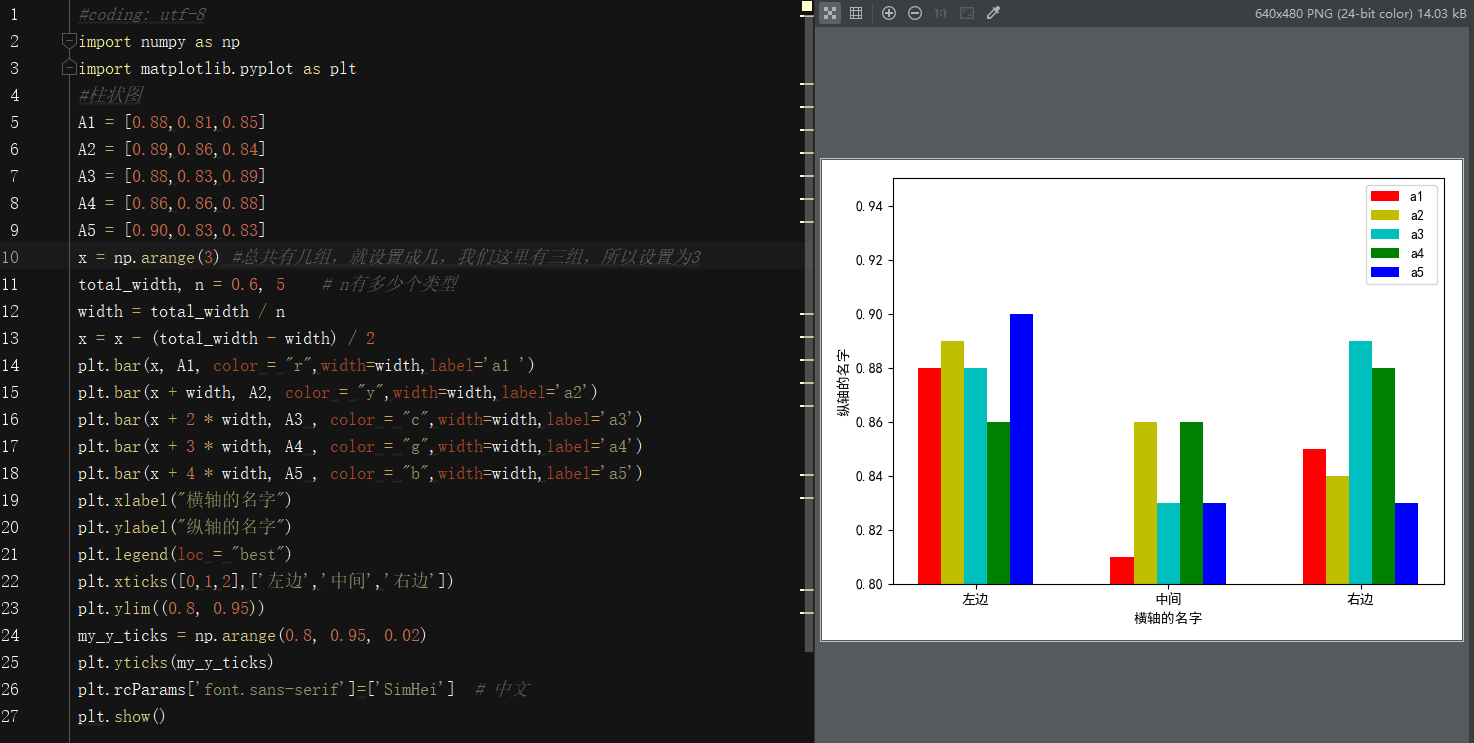#coding: utf-8
import numpy as np
import matplotlib.pyplot as plt
#柱状图
A1 = [0.88,0.81,0.85]
A2 = [0.89,0.86,0.84]
A3 = [0.88,0.83,0.89]
A4 = [0.86,0.86,0.88]
A5 = [0.90,0.83,0.83]
x = np.arange(3) #总共有几组，就设置成几，我们这里有三组，所以设置为3
total_width, n = 0.6, 5    # n有多少个类型
width = total_width / n
x = x - (total_width - width) / 2
plt.bar(x, A1, color = "r",width=width,label='a1 ')
plt.bar(x + width, A2, color = "y",width=width,label='a2')
plt.bar(x + 2 * width, A3 , color = "c",width=width,label='a3')
plt.bar(x + 3 * width, A4 , color = "g",width=width,label='a4')
plt.bar(x + 4 * width, A5 , color = "b",width=width,label='a5')
plt.xlabel("横轴的名字")
plt.ylabel("纵轴的名字")
plt.legend(loc = "best")
plt.xticks([0,1,2],['左边','中间','右边'])
plt.ylim((0.8, 0.95))
my_y_ticks = np.arange(0.8, 0.95, 0.02)
plt.yticks(my_y_ticks)
plt.rcParams['font.sans-serif']=['SimHei']  # 中文
plt.show()


## 2.3 饼图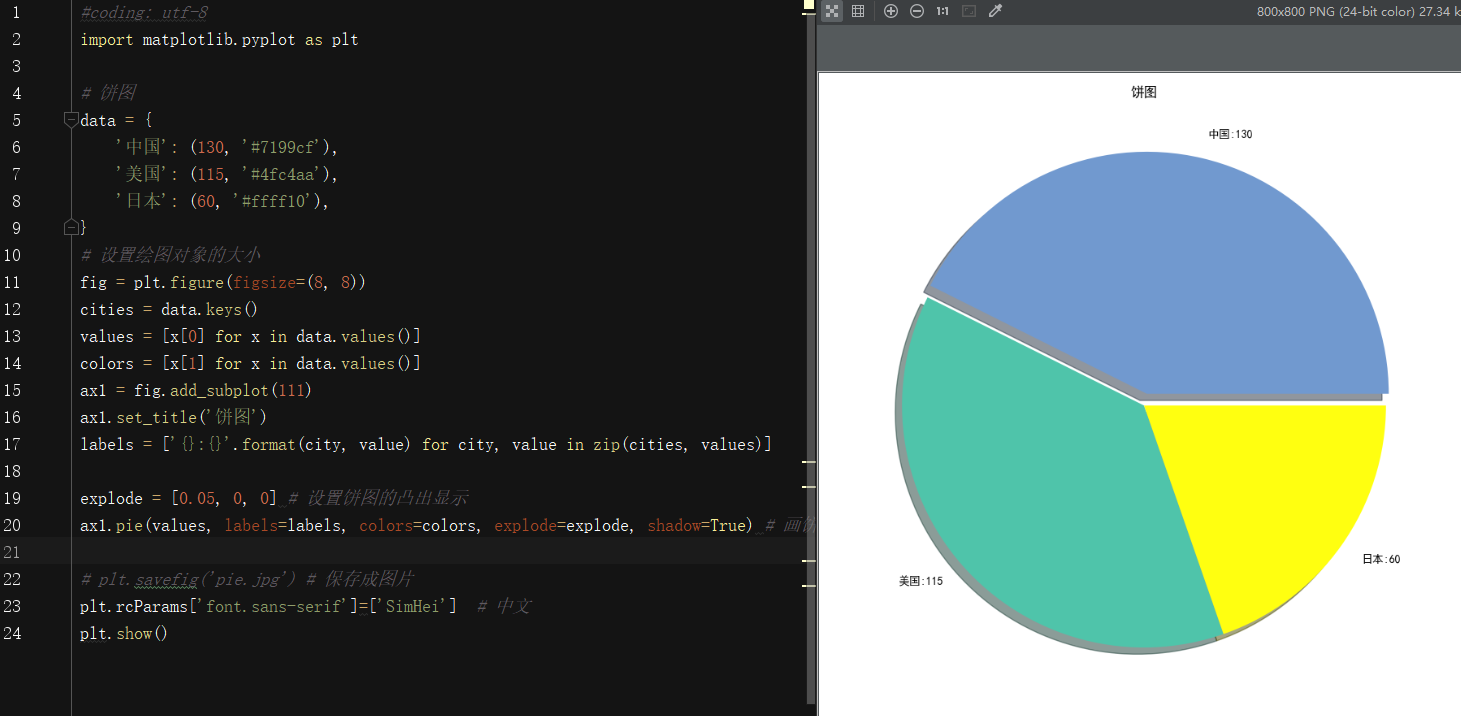#coding: utf-8
import matplotlib.pyplot as plt

# 饼图
data = {
'中国': (130, '#7199cf'),
'美国': (115, '#4fc4aa'),
'日本': (60, '#ffff10'),
}
# 设置绘图对象的大小
fig = plt.figure(figsize=(8, 8))
cities = data.keys()
values = [x for x in data.values()]
colors = [x for x in data.values()]
ax1.set_title('饼图')
labels = ['{}:{}'.format(city, value) for city, value in zip(cities, values)]

explode = [0.05, 0, 0] # 设置饼图的凸出显示
ax1.pie(values, labels=labels, colors=colors, explode=explode, shadow=True) # 画饼状图， 并且指定标签和对应的颜色  指定阴影效果

# plt.savefig('pie.jpg') # 保存成图片
plt.rcParams['font.sans-serif']=['SimHei']  # 中文
plt.show()


## 2.4 绘制三维散点图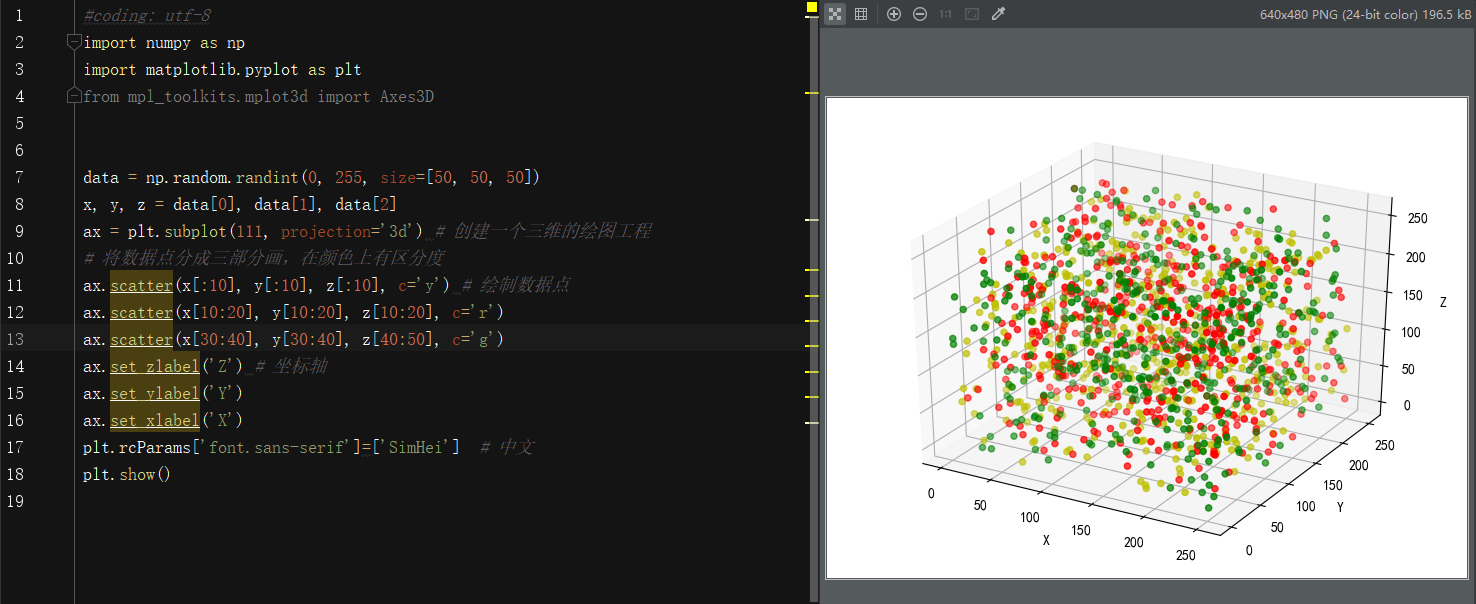#coding: utf-8
import numpy as np
import matplotlib.pyplot as plt
from mpl_toolkits.mplot3d import Axes3D

data = np.random.randint(0, 255, size=[50, 50, 50])
x, y, z = data, data, data
ax = plt.subplot(111, projection='3d') # 创建一个三维的绘图工程
# 将数据点分成三部分画，在颜色上有区分度
ax.scatter(x[:10], y[:10], z[:10], c='y') # 绘制数据点
ax.scatter(x[10:20], y[10:20], z[10:20], c='r')
ax.scatter(x[30:40], y[30:40], z[40:50], c='g')
ax.set_zlabel('Z') # 坐标轴
ax.set_ylabel('Y')
ax.set_xlabel('X')
plt.rcParams['font.sans-serif']=['SimHei']  # 中文
plt.show()


## 2.5 散点图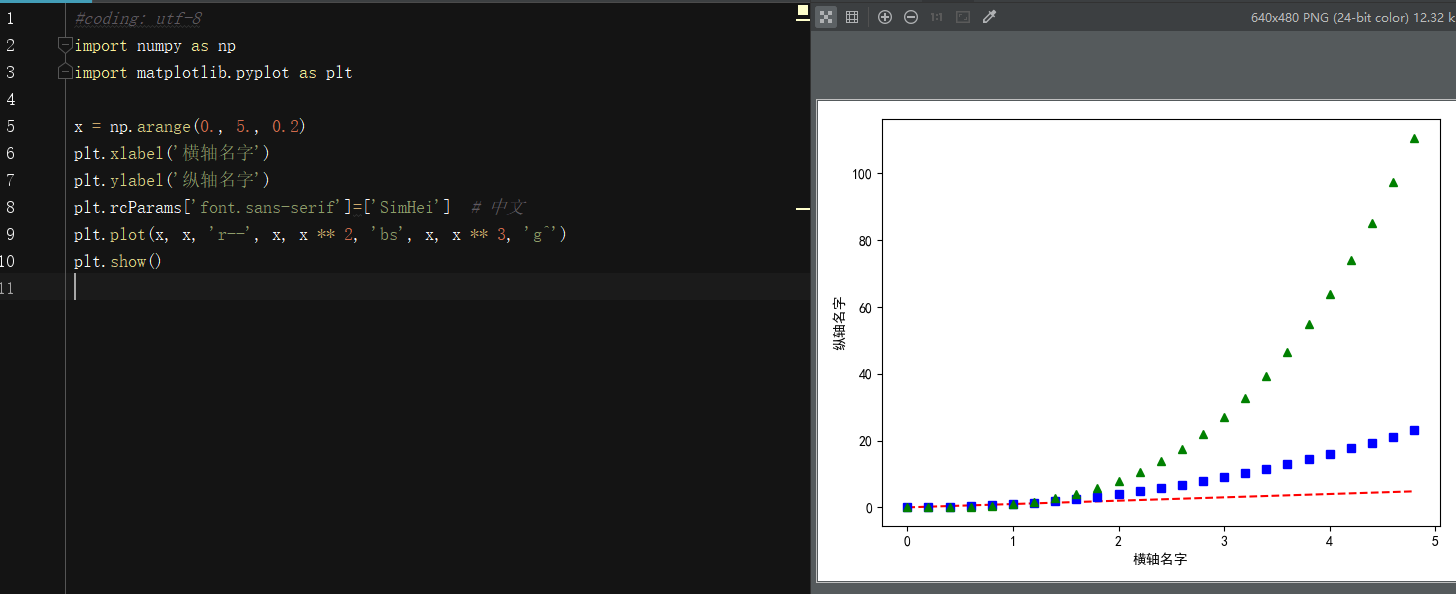#coding: utf-8
import numpy as np
import matplotlib.pyplot as plt

x = np.arange(0., 5., 0.2)
plt.xlabel('横轴名字')
plt.ylabel('纵轴名字')
plt.rcParams['font.sans-serif']=['SimHei']  # 中文
plt.plot(x, x, 'r--', x, x ** 2, 'bs', x, x ** 3, 'g^')
plt.show()



# 3、其它可视化库

## 3.1 Seaborn

Seaborn利用了matplotlib，用简洁的代码来制作好看的图表。Seaborn跟matplotlib最大的区别就是它的默认绘图风格和色彩搭配都具有现代美感。由于Seaborn是构建在matplotlib的基础上的，你需要了解matplotlib从而来调整Seaborn的默认参数。

## 3.2 ggplot

ggplot 基于R的一个作图包 ggplot2, 同时利用了源于 《图像语法》（The Grammar of Graphics）中的概念。ggplot 跟 matplotlib 的不同之处是它允许你叠加不同的图层来完成一幅图。比如你可以从轴开始，然后加上点，加上线，趋势线等等。虽然《图像语法》得到了“接近思维过程”的作图方法的好评，但是习惯了matplotlib的用户可能需要一些时间来适应这个新思维方式。ggplot的作者提到 ggplot 并不适用于制作非常个性化的图像。它为了操作的简洁而牺牲了图像复杂度。ggplot跟pandas的整合度非常高，所以当你使用它的时候，最好将你的数据读成 DataFrame。

## 3.3 Bokeh

Bokeh为不同的用户提供了三种控制水平。最高的控制水平用于快速制图，主要用于制作常用图像， 例如柱状图，盒状图，直方图。中等控制水平跟matplotlib一样允许你控制图像的基本元素（例如分布图中的点）。最低的控制水平主要面向开发人员和软件工程师。它没有默认值，你得定义图表的每一个元素。

## 3.4 pygal

pygal 跟 Bokeh 和 Plotly 一样，提供可直接嵌入网络浏览器的可交互图像。跟其他两者的主要区别在于它可以将图表输出为SVG格式。如果你的数据量相对小，SVG就够用了。但是如果你有成百上千的数据点，SVG的渲染过程会变得很慢。由于所有的图表都被封装成了方法，而且默认的风格也很漂亮，用几行代码就可以很容易地制作出漂亮的图表。

## 3.6 geoplotlib

geoplotlib 是一个用于制作地图和地理相关数据的工具箱。你可以用它来制作多种地图，比如等值区域图， 热度图，点密度图。你必须安装 Pyglet （一个面向对象编程接口）来使用geoplotlib。 不过因为大部分Python的可视化工具不提供地图，有一个专职画地图的工具也是挺方便的。
Github:https://github.com/andrea-cuttone/geoplotlib

## 3.7 Gleam

Gleam 借用了R中 Shiny 的灵感。 它允许你只利用 Python 程序将你的分析变成可交互的网络应用，你不需要会用HTML CSS 或者 JaveScript。Gleam 可以使用任何一种 Python 的可视化库。当你创建一个图表的时候，你可以在上面加上一个域，这样用户可以用它来对数据排序和过滤了。
Github:https://github.com/dgrtwo/gleam

## 3.8 missingno

Github: https://github.com/ResidentMario/missingno

## 3.9 Leather

Leather的最佳定义来自它的作者 Christopher Groskopf：“Leather 适用于现在就需要一个图表并且对图表是不是完美并不在乎的人。”它可以用于所以的数据类型然后生成SVG图像，这样在你调整图像大小的时候就不会损失图像质量。这个库很新，一些文档还没有最后完成。图像成品非常基础——但是这就是设计目标。

## 3.10 pastalog

Github: https://github.com/rewonc/pastalog

## 3.11 GazeParser

GazeParser是眼动研究的一个包。 对于录制，该软件包提供了一个模块来控制来自VisionEgg和PsychoPy的基于视频的开源眼动追踪应用程序SimpleGazeTracker。 对于数据分析，该软件包提供各种功能，例如检测扫视和固定，绘制和比较扫描路径，计算扫视轨迹曲率等。
pypi：https://pypi.org/project/GazeParser/0.11.1/©️2019 CSDN 皮肤主题: 代码科技 设计师: Amelia_0503